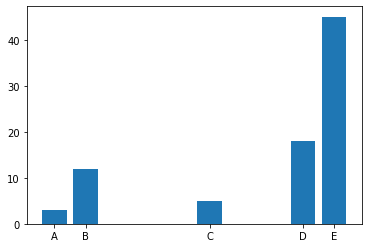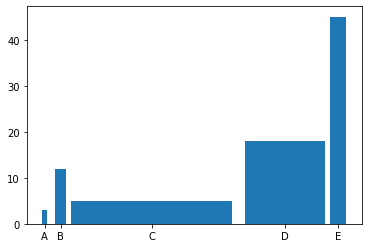## Control Space

In order to control the space between bars, you can specify the positions of bars in the x axis. This way, you will be able to control the spaces.

``````# library
import matplotlib.pyplot as plt

# create dataset
height = [3, 12, 5, 18, 45]
bars = ('A', 'B', 'C', 'D', 'E')

# Choose the position of each barplots on the x-axis (space=1,4,3,1)
x_pos = [0,1,5,8,9]

# Create bars
plt.bar(x_pos, height)

# Create names on the x-axis
plt.xticks(x_pos, bars)

# Show graphic
plt.show()``````## Control Width

The width of the bars can be controlled by `width` argument of the `bar()` function.

``````# library
import matplotlib.pyplot as plt

# create dataset
height = [3, 12, 5, 18, 45]
bars = ('A', 'B', 'C', 'D', 'E')

# Choose the width of each bar and their positions
width = [0.1,0.2,3,1.5,0.3]
x_pos = [0,0.3,2,4.5,5.5]

# Make the plot
plt.bar(x_pos, height, width=width)

# Create names on the x-axis
plt.xticks(x_pos, bars)

# Show graphic
plt.show()``````## Contact & Edit

👋 This document is a work by Yan Holtz. You can contribute on github, send me a feedback on twitter or subscribe to the newsletter to know when new examples are published! 🔥